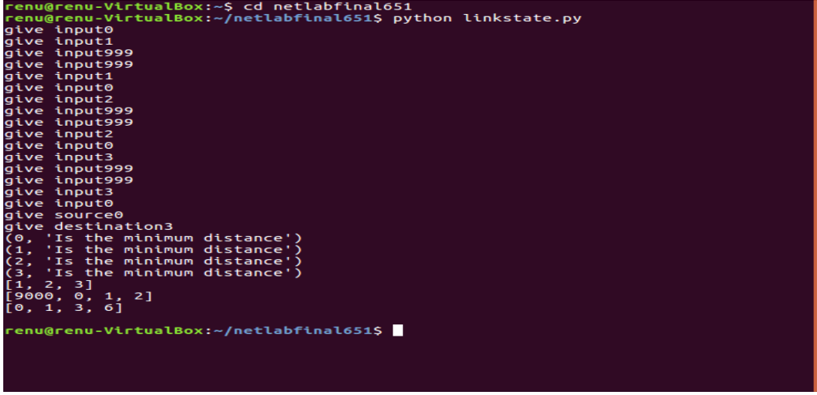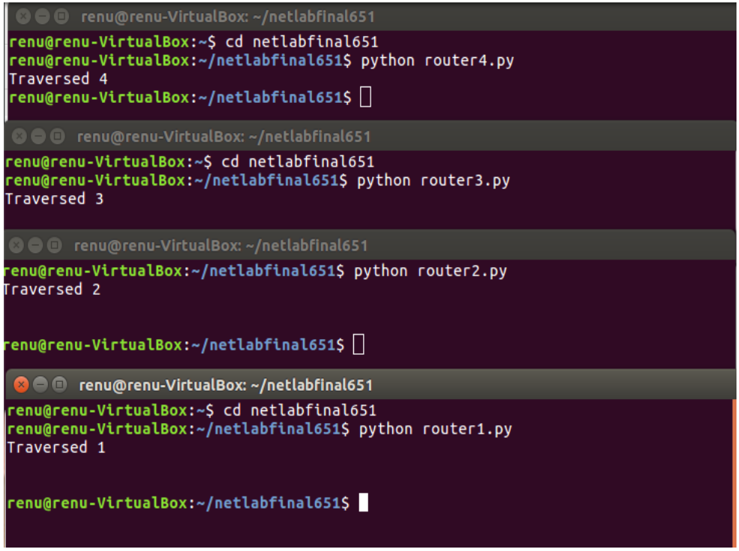Related Articles
Shortest Path Problem Between Routing Terminals – Implementation in Python
• Difficulty Level : Expert
• Last Updated : 27 Feb, 2020

The famous Dijkstra’s algorithm can be used in a variety of contexts – including as a means to find the shortest route between two routers, also known as Link state routing. This article explains a simulation of Dijkstra’s algorithm in which the nodes (routers) are terminals.
Once the shortest path between two nodes (terminals) is calculated, the shortest path itself is sent as a message to each terminal on its path in sequence, until the destination terminal is reached. Whenever the message has traversed a node, its terminal displays the traversal. In this way, it is possible to both see and simulate the passage of a message across a shortest calculated route.

The procedure to run the following code is as follows:

• Execute the driver code
• Before providing any input to the driver code, run the router codes router1.py, router2.py, etc. in separate terminals/tabs.
• Now provide input to the driver code in the form of a matrix G, in which any entry `G[i, j]` is the distance from node i to node j. The matrix must be symmetrical. If i=j, then` D[i, j]=0` as the distance between a node and itself is considered to be nothing. If there is no direct connection between two nodes, then `D[i, j]=999` (the equivalent of infinity).
• Specify source and destination nodes, where the nodes vary from 0 to 3, and represent terminals 1 to 4 respectively.

This implementation specifies four nodes but this can easily be extended to N nodes with N terminals and port numbers representing processes running on them respectively.

Consider the following example of a four-node network, with distances between them as specified and nodes numbered 0 to 3 from left to right:Distances between terminals

For this network, the matrix G with entries G[i, j] as specified above would be:

```[[0, 1, 999, 999],
[1, 0, 2, 999],
[999, 2, 0, 3],
[999, 999, 3, 0]]
```

This matrix would have to be input to the driver code. Dijkstra’s algorithm is used to find the shortest path between source and destination. A list containing the remaining path is sent to each node en route to the final destination.

The implementation in Python is specified below.

 `# Driver Code for implementing Dijkstra's algorithm``import` `socket``import` `sys``import` `pickle`` ` `S ``=` `set``() ``G ``=``[] ``# adjacency matrix`` ` `# give input matrix``for` `i ``in` `range``(``4``): ``    ``listo ``=``[``0``, ``0``, ``0``, ``0``]``     ` `    ``for` `j ``in` `range``(``4``):``        ``listo[j]``=` `int``(``input``(``"give input"``))``    ``G.append(listo)``     ` `source ``=` `int``(``input``(``"give source"``)) ``destination ``=` `int``(``input``(``"give destination"``)) ``Q ``=``[] ``# empty queue`` ` `for` `i ``in` `range``(``4``):``    ``Q.append(i)``     ` `d ``=``[``0``, ``0``, ``0``, ``0``] ``# initialize d values``pi ``=``[``0``, ``0``, ``0``, ``0``] ``# initialize pi values`` ` `for` `i ``in` `range``(``4``):``    ``if``(i ``=``=` `source):``        ``d[i]``=` `0``    ``else``:``        ``d[i]``=` `999``for` `i ``in` `range``(``4``):``    ``pi[i]``=` `9000``S.add(source)`` ` `# While items still exist in Q``while` `(``len``(Q)!``=` `0``): ``     ` `    ``# Find the minimum distance x from``    ``# source of all nodes in Q``    ``x ``=` `min``(d[q] ``for` `q ``in` `Q) ``    ``u ``=` `0``    ``for` `q ``in` `Q:``        ``if``(d[q]``=``=` `x):``             ` `            ``# Find the node u in Q with minimum ``            ``# distance x from source ``            ``u ``=` `q ``             ` `    ``print``(u, ``"Is the minimum distance"``)``    ``Q.remove(u) ``# removed the minimum vertex``    ``S.add(u)``    ``adj ``=``[]``    ``for` `y ``in` `range``(``4``):``         ` `        ``# find adjacent vertices to minimum vertex``        ``if``(y !``=` `u ``and` `G[u][y]!``=` `999``):     ``            ``adj.append(y)``             ` `     ``# For each adjacent vertex, perform the update``     ``# of distance and pi vectors        ``    ``for` `v ``in` `adj:        ``        ``if``(d[v]>(d[u]``+``G[u][v])):``            ``d[v]``=` `d[u]``+``G[u][v] ``            ``pi[v]``=` `u ``# update adjacents distance and pi``route ``=``[]``x ``=` `destination`` ` `# If destination is source, then pi[x]= 9000. ``if``(pi[x]``=``=` `9000``): ``    ``print``(source)``else``:``     ` `    ``# Find the path from destination to source``    ``while``(pi[x]!``=` `9000``): ``        ``route.append(x)``        ``x ``=` `pi[x]``    ``route.reverse() ``     ` `     ` `print``(route) ``# Display the route``print``(pi) ``# Display the path vector``print``(d) ``# Display the distance of each node from source`` ` `'''We will now send the calculated minimal route to the terminal ``# representing 'source'. From the source terminal, the 'route' list ``# will be sent to the next hop en route to the final destination. `` ` `# At each intermediate terminal, the router removes its own identity `` ``from the list and sends the rest of the route to the next router. `` ``This continues until the final router is reached.'''``  ` `sendingroute ``=` `pickle.dumps(route)``sockets ``=``[``8895``, ``8896``, ``8897``, ``8898``]``sock ``=` `socket.socket(socket.AF_INET, socket.SOCK_STREAM) ``sock.connect((socket.gethostname(), sockets)) `` ` `try``:``     ` `    ``# try sendall if it doesn't work. ``    ``sock.send(sendingroute) ``finally``:``    ``print``("")``sock.close()`
 `# Code for Router 1``import` `socket``import` `sys``import` `pickle`` ` `for` `i ``in` `range``(``1``) :``    ``sock ``=` `socket.socket(socket.AF_INET, socket.SOCK_STREAM)``    ``sock.bind((socket.gethostname(), ``8895``))``    ``sock.listen(``1``)``    ``connection, client_address ``=` `sock.accept()``    ``route ``=``[]``    ``sockets ``=``[``8895``, ``8896``, ``8897``, ``8898``]`` ` `    ``while` `1``:``        ``try``:``            ``route ``=` `pickle.loads(connection.recv(``1024``))``    ``except` `EOFError:``        ``break`      `        ``finally``:``            ``break``    ``print``(``"Traversed 1"``) ``    ``socknext ``=` `socket.socket(socket.AF_INET, socket.SOCK_STREAM)`` ` `    ``if``(``len``(route)>``0``):``        ``x ``=` `route[``0``]``        ``route.remove(x)``        ``dataroute ``=` `pickle.dumps(route)``        ``socknext.connect((socket.gethostname(), sockets[x]))``        ``try``:``            ``socknext.send(dataroute) ``# try sendall``            ``data ``=` `socknext.recv(``16``)``            ``print``(data)``       ``finally``:``               ``print``("")``        ``socknext.close()`

 `# Code for Router 2``import` `socket``import` `sys``import` `pickle`` ` `for` `i ``in` `range``(``1``) :`` ` `    ``sock ``=` `socket.socket(socket.AF_INET, socket.SOCK_STREAM)``    ``sock.bind((socket.gethostname(), ``8896``))``    ``sock.listen(``1``)``    ``connection, client_address ``=` `sock.accept()``    ``route ``=``[]``    ``sockets ``=``[``8895``, ``8896``, ``8897``, ``8898``]`` ` `    ``while` `1``:``        ``try``:``            ``route ``=` `pickle.loads(connection.recv(``1024``))``    ``except` `EOFError:``        ``break`      `        ``finally``:``            ``break``    ``print``(``"Traversed 2"``)`` ` `    ``socknext ``=` `socket.socket(socket.AF_INET, socket.SOCK_STREAM)`` ` `    ``if``(``len``(route)>``0``):`` ` `    ``x ``=` `route[``0``]``        ``route.remove(x)``        ``dataroute ``=` `pickle.dumps(route)``        ``socknext.connect((socket.gethostname(), sockets[x]))``        ``try``:``            ``socknext.send(dataroute) ``# try sendall``            ``data ``=` `socknext.recv(``16``)``            ``print``(data)``       ``finally``:``               ``print``("")``        ``socknext.close()`
 `# Code for Router 3``import` `socket``import` `sys``import` `pickle`` ` `for` `i ``in` `range``(``1``) :``    ``sock ``=` `socket.socket(socket.AF_INET, socket.SOCK_STREAM)``    ``sock.bind((socket.gethostname(), ``8897``))``    ``sock.listen(``1``)``    ``connection, client_address ``=` `sock.accept()`` ` `    ``route ``=``[]``    ``sockets ``=``[``8895``, ``8896``, ``8897``, ``8898``]``    ``while` `1``:``        ``try``:``            ``route ``=` `pickle.loads(connection.recv(``1024``))``    ``except` `EOFError:``        ``break`      `        ``finally``:``            ``break``    ``print``(``"Traversed 3"``)``    ``socknext ``=` `socket.socket(socket.AF_INET, socket.SOCK_STREAM)`` ` `    ``if``(``len``(route)>``0``):``    ``x ``=` `route[``0``]``        ``route.remove(x)``        ``dataroute ``=` `pickle.dumps(route)``        ``socknext.connect((socket.gethostname(), sockets[x]))``        ``try``:``            ``socknext.send(dataroute) ``# try sendall``            ``data ``=` `socknext.recv(``16``)``            ``print``(data)``       ``finally``:``               ``print``("")``        ``socknext.close()`
 `# Code for Router 4``import` `socket``import` `sys``import` `pickle`` ` `for` `i ``in` `range``(``1``) :``    ``sock ``=` `socket.socket(socket.AF_INET, socket.SOCK_STREAM)``    ``sock.bind((socket.gethostname(), ``8898``))``    ``sock.listen(``1``)``    ``connection, client_address ``=` `sock.accept()``    ``route ``=``[]``    ``sockets ``=``[``8895``, ``8896``, ``8897``, ``8898``]`` ` `    ``while` `1``:``        ``try``:``            ``route ``=` `pickle.loads(connection.recv(``1024``))``    ``except` `EOFError:``        ``break`      `        ``finally``:``            ``break`` ` `    ``print``(``"Traversed 4"``)``    ``socknext ``=` `socket.socket(socket.AF_INET, socket.SOCK_STREAM)`` ` `    ``if``(``len``(route)>``0``):``    ``x ``=` `route[``0``]``        ``route.remove(x)``        ``dataroute ``=` `pickle.dumps(route)``        ``socknext.connect((socket.gethostname(), sockets[x]))``        ``try``:``            ``socknext.send(dataroute) ``# try sendall``            ``data ``=` `socknext.recv(``16``)``            ``print``(data)``       ``finally``:``               ``print``("")``        ``socknext.close()`Dijkstra Output

Terminal Output –Terminal Output

Attention geek! Strengthen your foundations with the Python Programming Foundation Course and learn the basics.

To begin with, your interview preparations Enhance your Data Structures concepts with the Python DS Course.

My Personal Notes arrow_drop_up